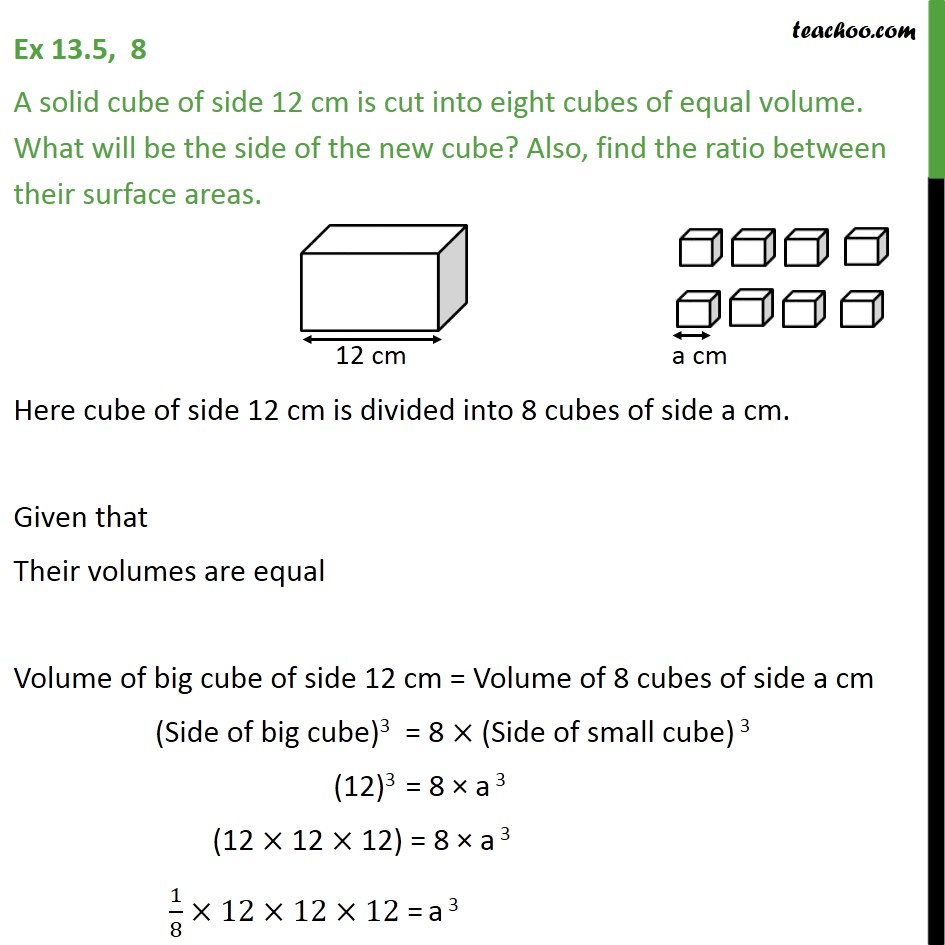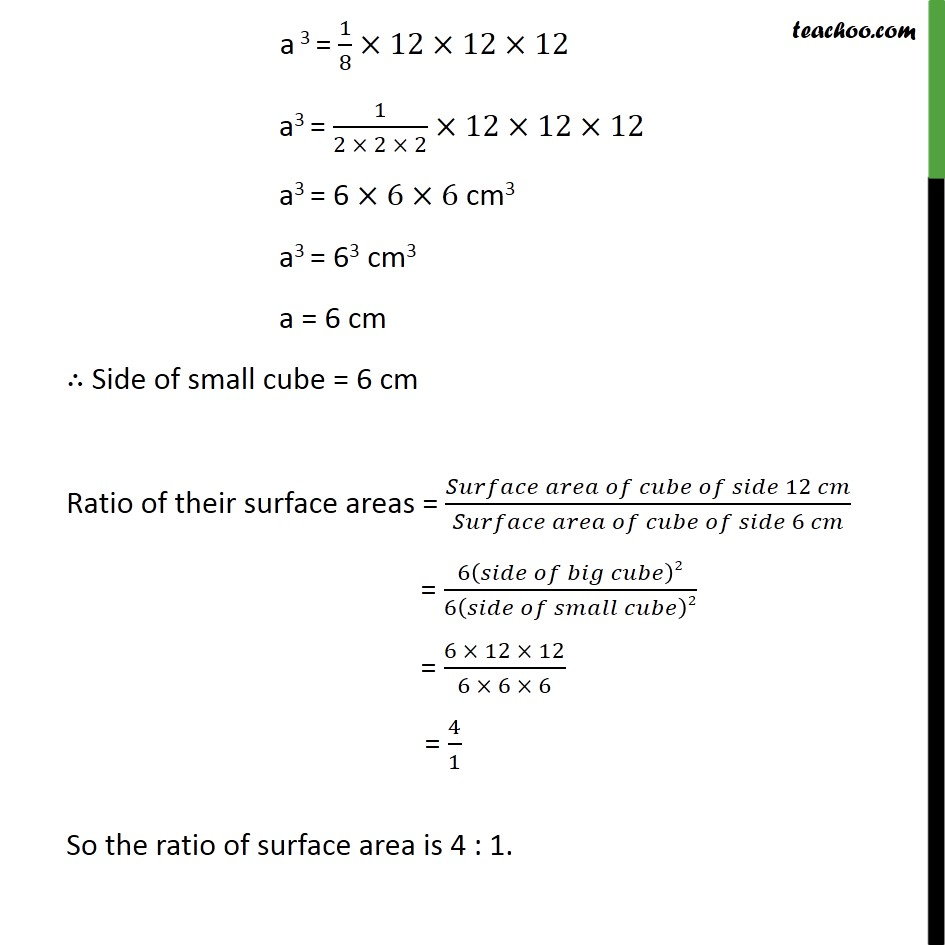Chapter 13 Class 9 Surface Areas and Volumes

Class 9
Important Questions for Exam - Class 9Learn in your speed, with individual attention - Teachoo Maths 1-on-1 Class

### Transcript

Question 8 A solid cube of side 12 cm is cut into eight cubes of equal volume. What will be the side of the new cube? Also, find the ratio between their surface areas. Here cube of side 12 cm is divided into 8 cubes of side a cm. Given that Their volumes are equal Volume of big cube of side 12 cm = Volume of 8 cubes of side a cm (Side of big cube)3 = 8 × (Side of small cube) 3 (12)3 = 8 × a 3 (12 × 12 × 12) = 8 × a 3 1/8×12×12×12 = a 3 a 3 = 1/8×12×12×12 a3 = 1/(2 × 2 × 2)×12×12×12 a3 = 6 ×6×6 cm3 a3 = 63 cm3 a = 6 cm ∴ Side of small cube = 6 cm Ratio of their surface areas = (𝑆𝑢𝑟𝑓𝑎𝑐𝑒 𝑎𝑟𝑒𝑎 𝑜𝑓 𝑐𝑢𝑏𝑒 𝑜𝑓 𝑠𝑖𝑑𝑒 12 𝑐𝑚)/(𝑆𝑢𝑟𝑓𝑎𝑐𝑒 𝑎𝑟𝑒𝑎 𝑜𝑓 𝑐𝑢𝑏𝑒 𝑜𝑓 𝑠𝑖𝑑𝑒 6 𝑐𝑚) = 6(𝑠𝑖𝑑𝑒 𝑜𝑓 𝑏𝑖𝑔 𝑐𝑢𝑏𝑒)2/6(𝑠𝑖𝑑𝑒 𝑜𝑓 𝑠𝑚𝑎𝑙𝑙 𝑐𝑢𝑏𝑒)2 = (6 × 12 × 12)/(6 × 6 × 6) = 4/1 So the ratio of surface area is 4 : 1.